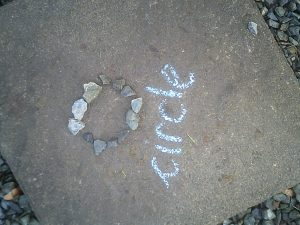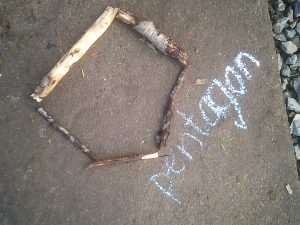## Maths Homework

This weekends Maths Challenge to be  completed by Monday 14th December

Triongl/Sgwar

Ask your grown-up to tell you a number up to 20.  Place this number of raisins/cereal pieces on your plate. If your grown-up says ‘one more’, can you add one more and say the number you have? If your grown-up says ‘one less’, can you eat one then count the number you have left?

Can you either email some of you number sentences that you made to your class teacher or email a photograph of you completing the activity to your teacher.

Pentagon/Cylch

Add ‘9’, ’19’, and 29 to the following numbers…..27, 36, 48, 52, 68, 72, 83, 94.

Remember to use the strategy that we practised in class.  For example, when adding 19, you would add 20 and then take away 1.

So, 34 + 19 you would do 34 + 20 = 54 and then 54-1 = 53.

Therefore, 34 + 19 = 53

Thanks

## Maths Homework

Happy Friday! This weekends homework is a Maths worksheet based on the work that the children have done in class this week.  Hard copies have been given to all children today and will need to be completed by Monday. Thanks

## Pupil Voice-Curriculum Planning Spring 2021

Today, we were visited by two little pigs and unfortunately the Big Bad Wolf! The pigs needed help to design a strong house that would save them from the wolf.  As next term, our topic is Houses and Homes, we also thought about what we would like to learn about and we mind-mapped our ideas!

## You will not need to email or upload evidence child learning these tables.

Triongl –  Please learn to count up in 10’s to 100

Sgwar – Please learn to count up in 2’s to 20.

Cylch – Please practise your 2’s, 3’s, 5’s and 10 times tables.

Thanks

## Maths Homework for Monday 23rd November

This weeks Maths Challenge is for your child to learn their number bonds.  You will not need to email or upload evidence this week of your child learning these bonds as we will be assessing them on their knowledge on Monday 23rd November.

Many Thanks

Triongl Group –  Learn your number bonds (pairs) to 5.

0 + 5 = 5

1 + 4 = 5

2 + 3 = 5

3 + 2 = 5

4 + 1 = 5

5 + 0 = 5

Sgwar Group –  Learn your number bonds (pairs) to 10.

0 + 10 = 10

1 + 9 = 10

2 + 8 = 10

3 + 7 = 10

4 + 6 = 10

5 + 5 = 10

6 + 4 = 10

7 + 3 = 10

8 + 2 = 10

9 + 1 = 10

10 + 0 = 10

Pentagon and Cylch Group –  Learn your number bonds (pairs) to 20.

0 + 20 = 20

1 + 19 = 20

2 + 18 = 20

3 + 17 = 20

4 + 16 = 20

5 + 15 = 20

6 + 14 = 20

7 + 13 = 20

8 + 12 = 20

9 + 11 = 20

10 + 0 = 20

11 + 9 = 20

12 + 8 = 20

13 + 7 = 20

14 + 6 = 20

15 + 5 = 20

16 + 4 = 20

17 + 3 = 20

18 + 2 = 20

19 + 1 = 20

20 + 0 = 20

## Eryr and Gwennol Maths Homework

Task for children in groups Triongl/Sgwar

Use natural objects to make the following shapes….triangles, circles, squares and rectangles.

Here is an example:Task for children in groups Pentagon/CylchUse natural objects to make the following shapes….pentagons, hexagons and octagons.  They can be regular or irregular.

Here is an example: